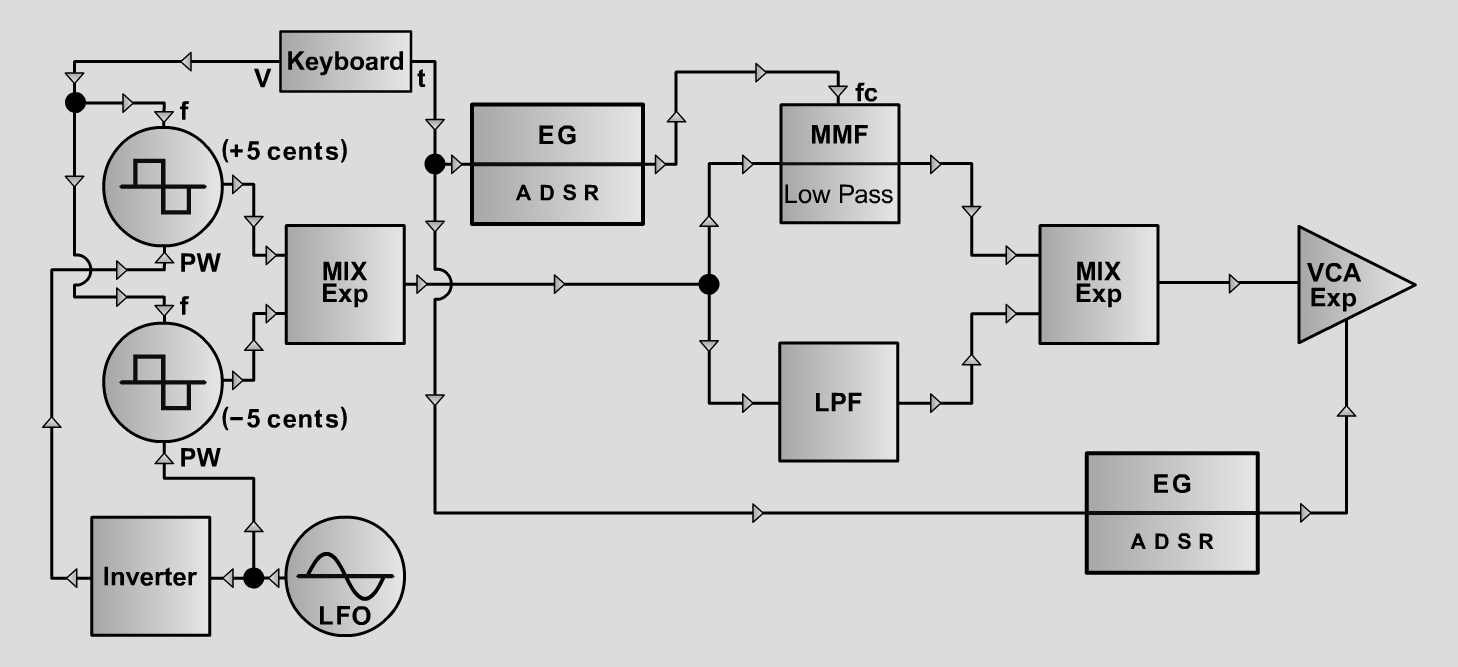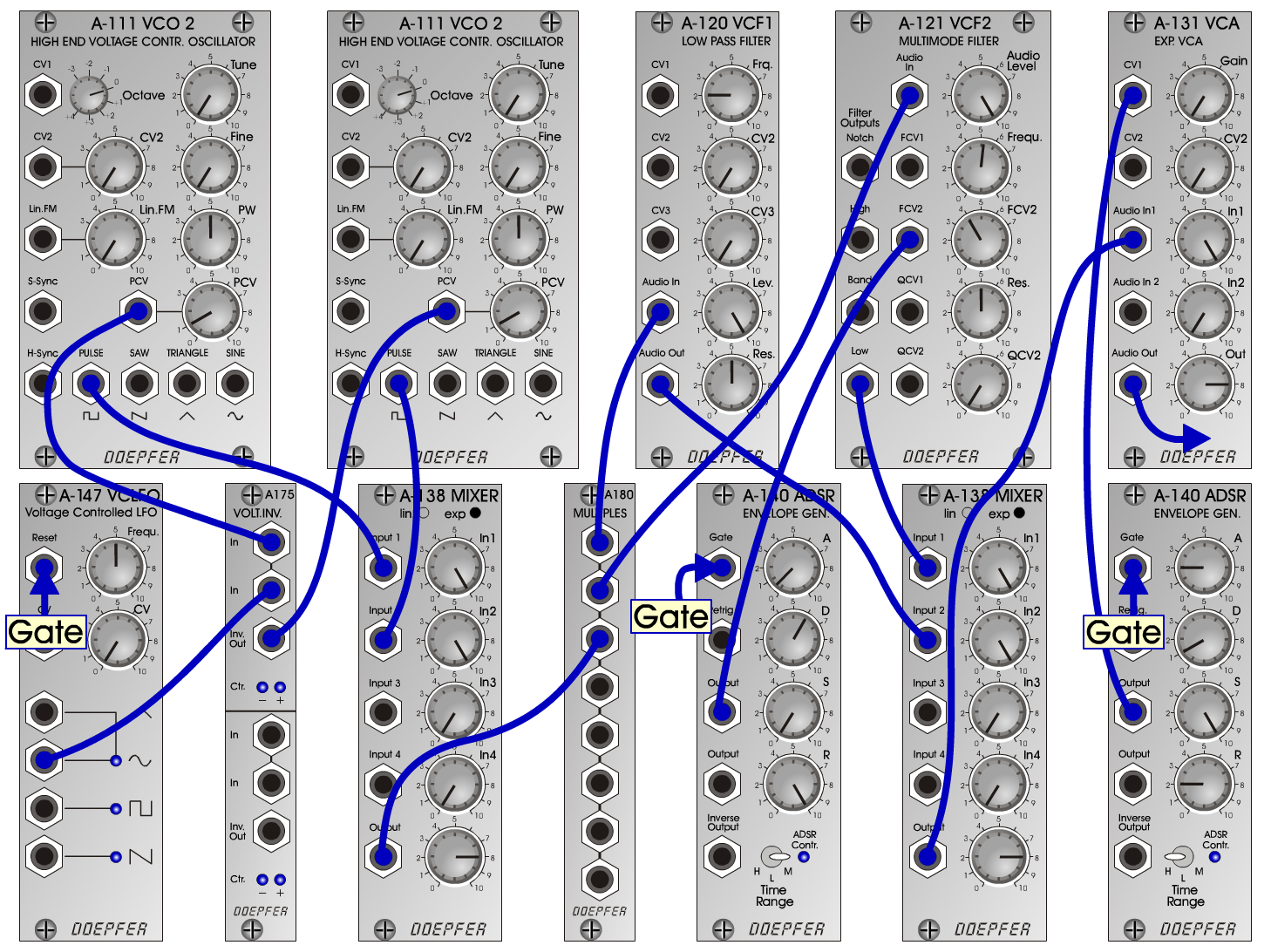StringPadS y n t h- P a d>D o e p f e r A - 100 Connections: Settings: A-111/1 (Square) <=> A-138exp/1 (Input 1)  A-111/2 (Square) <=> A-138exp/1 (Input 2)  A-138exp/1 (Output) <=> A-180 (1)   A-180 (2) <=> A-120 (Audio In)  A-180 (3) <=> A-121 (Audio In)  A-120 (Audio Out) <=> A-138exp/2 (Input 1)  A-121 (Low) <=> A-138exp/2 (Input 2)  A-138exp/2 (Output) <=> A-131 (Audio In)  A-147 (Sine) <=> A-175 (In-1)  A-175 (In-2) <=> A-111/1 (PCV)  A-175 (Inv Out) <=> A-111/2 (PCV)  A-140/1 (Output) A-131 (CV1)<=>  A-140/2 (Output) <=> A-121 (FCV2)  Gate <=> A-147 (Reset) A-111/1 (PW = 5, PCV = 1)  A-111/2 (PW = 5, PCV = 1)  A-120 (Freq = 2, Lev = 10, Res = 5)  A-121 (Audio Level = 10, Freq = 5.2, FCV 2 = 4, Res = 5)  A-140/1 (A = 2, D = 1, S = 10, R = 2, Range = H)  A-140/2 (A = 0.5, D = 6, S = 0, R = 10, Range = M)  A-138exp/1 (In 1 = 10, In 2 = 10, Out = 8)  A-138exp/2 (In 1 = 10, In 2 = 10, Out = 8)  A-147 (Freq = 5  A-131 (Gain = 0, Audio In 1 = 10, Audio Out = 8) Tuning: A-111/1 => +5 cents; A-111/2 => -5 cents; Josef MuellerSound samples StringPad Piece StringPad Piece (with reverb) StringPad A.mp3 StringPad B.mp3 StringPad C.mp3